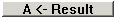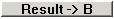# Subtract Vectors

Two vectors with the same dimension can be subtracted subtracting the first components, subtracting the second components, subtracting the third components and so on. The result is a vector with the same dimension as the original vectors.

Enter the two vectors and click thebutton in the middle of the window. The result appears in the middle of the window at the bottom.

You can do calculations with the result by moving the answer to the left sideor to the right side.

You cannot subtract vectors with different dimensions.